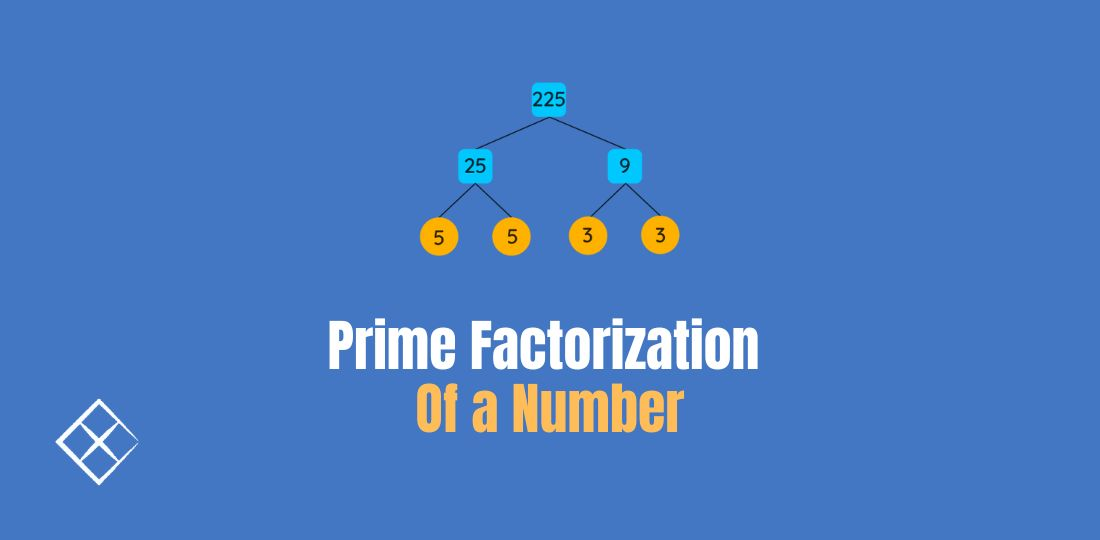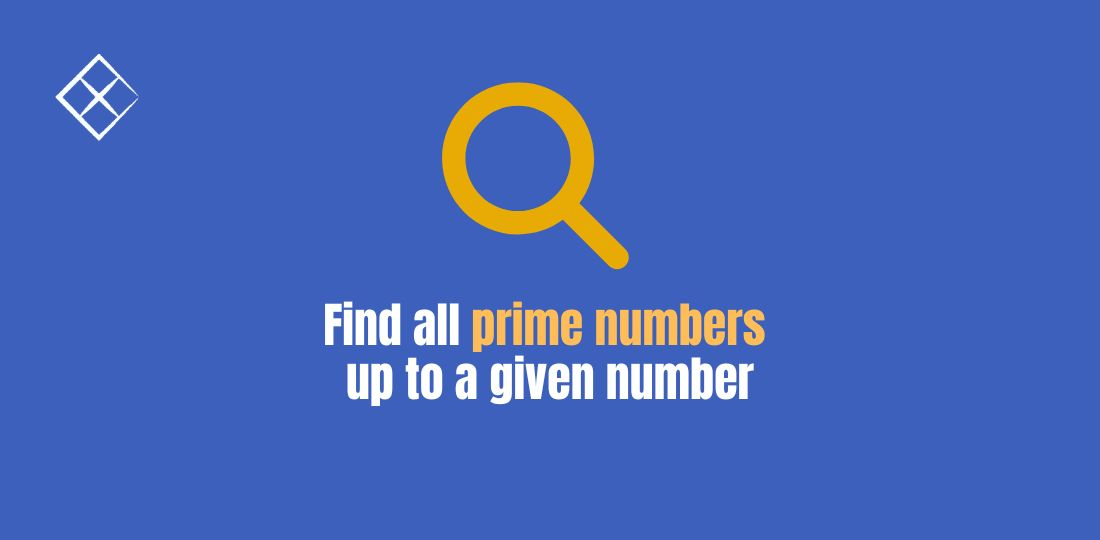# Showing items from Number Theory### Prime Factorization of a Number

Problem Statement How do we find all the prime factors of a given number? For example, when n = 12, …### Find all prime numbers up to a given number

Problem Statement How do we find all the prime numbers up to a given number? For example, when n = …